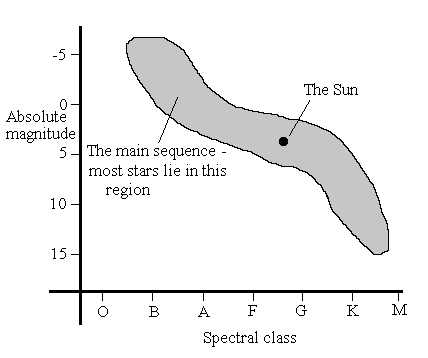Temperature and Luminosity

We can now work out a star's temperature simply by looking at it, but how does this help us calculate its distance? The answer is Stefan's Law . This equation relates a star's surface temperature to its luminosity . The only problem is that we must know the star's surface area to do the calculation. It is often necessary to assume that stars of the same type have similar sizes. Once we know the luminosity, the absolute magnitude can be found and so the distance. (Click here to find out about the magnitude system).The way this method works is displayed well by plotting absolute magnitude of stars against their spectral type . This graph (above), known as a Hertzsprung-Russell diagram shows that most stars lie on a straight line - the main sequence .